# Constrained Reinforcement Learning and self driving cars – An intern’s journey

This blog was written by one of our interns, Max Brodeur, and describes his summer project!

## Intro

With Reinforcement Learning (RL), we can teach complex tasks to computers by positively and negatively reinforcing good and bad behaviours. For example, in the context of video games, we can teach an RL model to navigate complex 3D maps like a player would. This technology has the potential to change the way we make our games, from improving our testing capabilities, all the way to having cool bots in front of players.

However, setting up a good RL training proves to be a difficult task. In particular, the reward shaping process is one of its hindering aspects. The behaviour specification framework developed at Ubisoft La Forge aims to alleviate the reward shaping problem by simplifying the behavior metrics and automating the weighting process.

Building upon this work, we set out to generalize the implementation and apply it to the problem of vehicle navigation in video games. After a summer internship project, we demonstrated that our generalized implementation greatly simplifies the reward shaping process. This is a promising approach to improve the productivity of game developers.

In this blog post, we provide a brief overview of the vehicle navigation problem, the reward shaping process, and Constrained RL theory (if you want to know more about this, you should definitely look into the paper or this blog post). Then, we showcase the vehicle navigation with behaviour specification — with cool videos!

There are many uses for a vehicle-operating RL model, such as game testing, where game designers can launch models on a variety of paths and verify their feasibility. For this project, we train vehicles in an environment that contains a variety of different terrains which range in difficulty and geometry.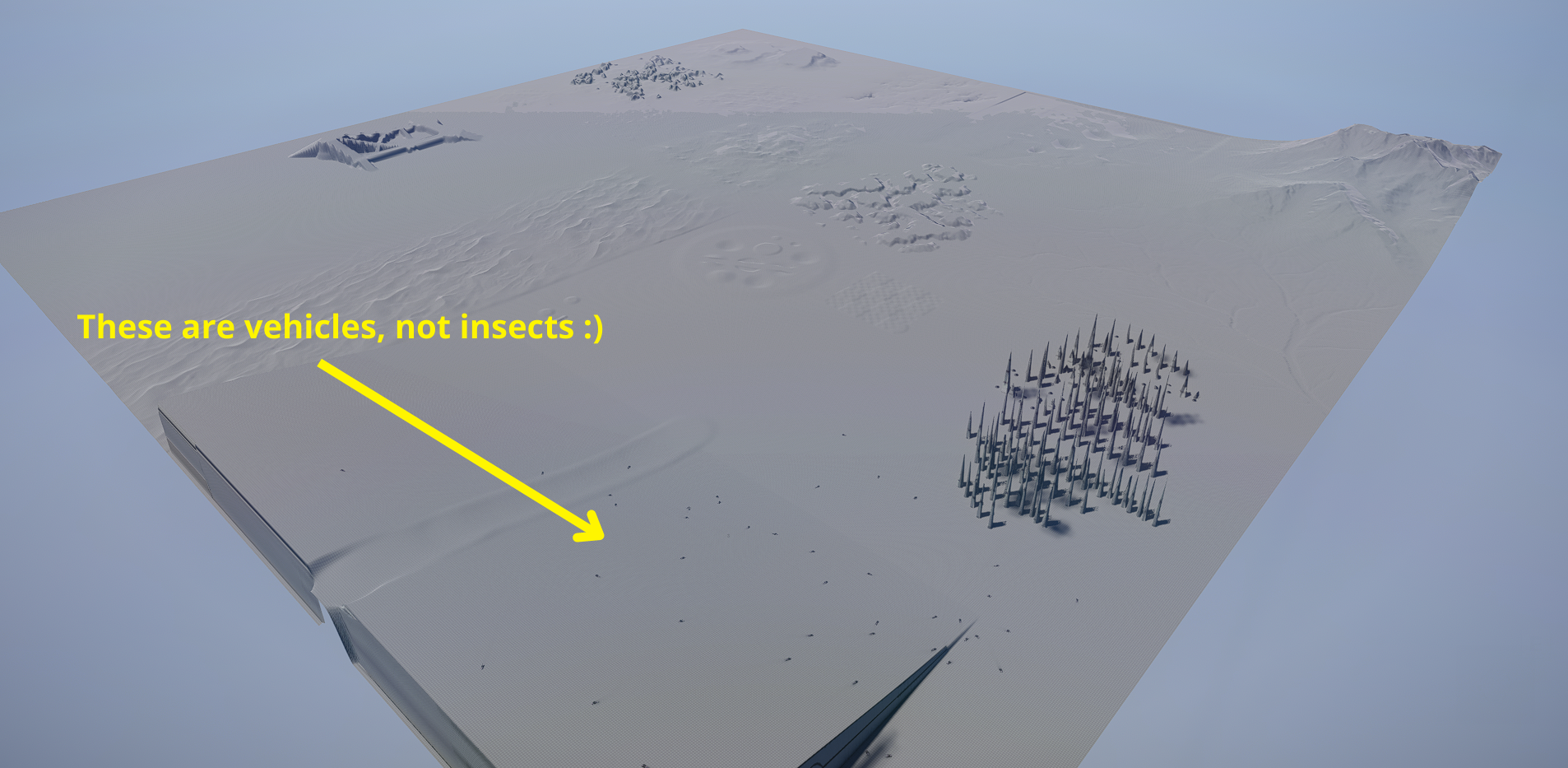The vehicles are spawned at arbitrary locations in the map and are given randomly generated paths and target speeds. Their task consists of navigating to the end of the path, at the correct speed. The model is given enough environment information to “understand” the problem, such as the distance from the path, elevation angles, obstacle distances, etc.Before we dig into our approach, lets discuss the classical approach to solving this problem with RL using reward shaping.

## Reward Shaping

Reward shaping consists of carefully crafting a mathematical function to evaluates the success of an action our model has taken. The reward is somewhat equivalent to a tasty doggie treat after a successful cool trick, or a dire “bad dog!” after an episode failure.

F(x)= Doggie Treat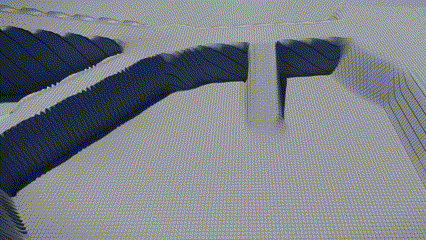It is easy to imagine how complex this mathematical function can get for some tasks. The example of self-driving vehicles in video games is one that requires a substantial reward function. In fact, one reward function I saw at Ubisoft took almost 400 lines of code.

First, let’s establish the concept of a reward function. It provides a way to specify a desired behavior to the model by evaluating the success of an action. For the vehicle navigation problem, we want the model to drive on the provided path at a certain target speed. For these behaviors, we will define the following reward function: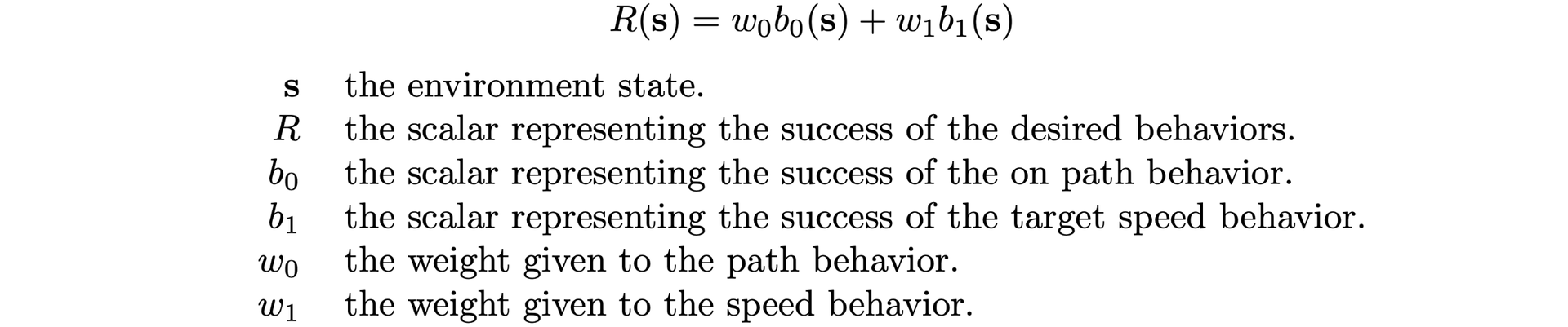In general, the goal of an RL training is to maximize the expected value of the sum of future rewards. Now that we have a function, we can better define the notion of reward shaping. Simply put, reward shaping is the tweaking of the reward function’s components. It consists of finding the best metrics b0 & b1 to express the desired behaviors, while finding the best weights w0 & w1 to apply to these metrics.

A common situation which necessitates the weighting of behaviors is the following: Let’s assume we launch an unweighted training (i.e. w0 = 1 & w1 =1). Upon initialization, the vehicle is already on the path, but is definitely not driving at the correct target speed. Due to the difference in frequency of these two behaviors, the model is likely to concentrate too much on one, while neglecting the other (which depends on the chosen sign of their reward). A knowledgeable developer would take into account these caveats, and immediately construct a reward function with less weight on the most frequent behavior. However, with increasingly complex tasks, an observation of the model’s performance is often required to determine how to change the weighting. In this case, our vehicle navigation problem can require over a dozen hours of training before yielding significant performances. In the end, unless you got lucky, some reward shaping will almost always be required to get the best results, which could take days, or even weeks!

## Constrained Reinforcement Learning

Our Constrained RL framework was developed to alleviate the reward shaping process and hopefully lead to a solution which requires no further parameter tweaking. Instead of providing a reward as a single value through a well-crafted reward function, our framework automatically weights binary behaviors.

For the sake of automating the weighting process, it is difficult to mathematically interpret behavior values when they are expressed as continuous scalars. For example, how should we interpret the following values?Is 0.5 good or bad? We would need some knowledge of what their behavior corresponds to in the environment. The key step in the research was to express the behaviors as binary values. This provides a format to interpret behavior values, regardless of their context in the environment. We define a binary constraint to be a behavior expressed in the following manner:The negation in this definition is by design – the implementation aims to minimize the constraint occurrences. Indeed, when they’re expressed as binary constraints, all behavior values have the same meaning, and therefore we can easily compare them.

But how do we compare them?

This is where it all comes together. Consider constraint values for N time steps. We can evaluate the constraint performances with the following definition: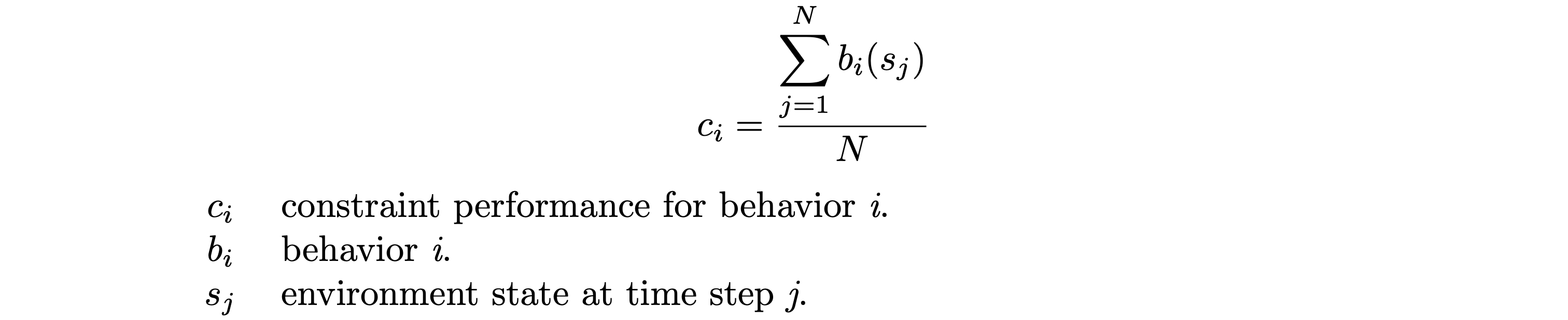Which is really just a fancy way of defining the percentage of time the behavior is occurring in a sample. We can now compare constraints in terms of their performances, i.e., ci = 30% indicates the behavior bioccurred 30% of the time in your sampled batch.

What about automating the weighting of the constraints? To solve this, we need thresholds. For each constraint ci we define a threshold tiwhich represents a target performance. As we know, for each binary constraint bi we have a weight wi. Using some fancy ML gradient descent tools (Adam Optimization for this implementation), we minimize the following error function with respect to the wi weight dimensions:The weights are passed through a softmax layer. Gradient descent through a softmax layer results in the weight update being relative to the magnitude of the other weights, which proves to be useful for assigning priority to poorly performing constraints.

The final component required as input for the Constrained RL implementation (along with binary constraints and thresholds) is the sparse reward. Unlike the constraints, training will maximize the expected value of the sparse reward.An extra weight wr is added to the weight vector for the sparse reward. However, we let Err( wr ) = 0 for all weight updates. This way, the sparse reward’s weight update will only depend on the softmax gradient. Thus, the weight will only increase once the other weights have decreased enough. In other words, once the constraints are satisfied, the training concentrates on maximizing the sparse reward.

Instead of a scalar value, the reward is now a multidimensional vector with the binary constraints and the sparse reward as values. This reward is computed at every time step during training. Along with the threshold vector, this is the only information needed for training.## Results

Consider the following configuration with the previously defined path and speed behaviors: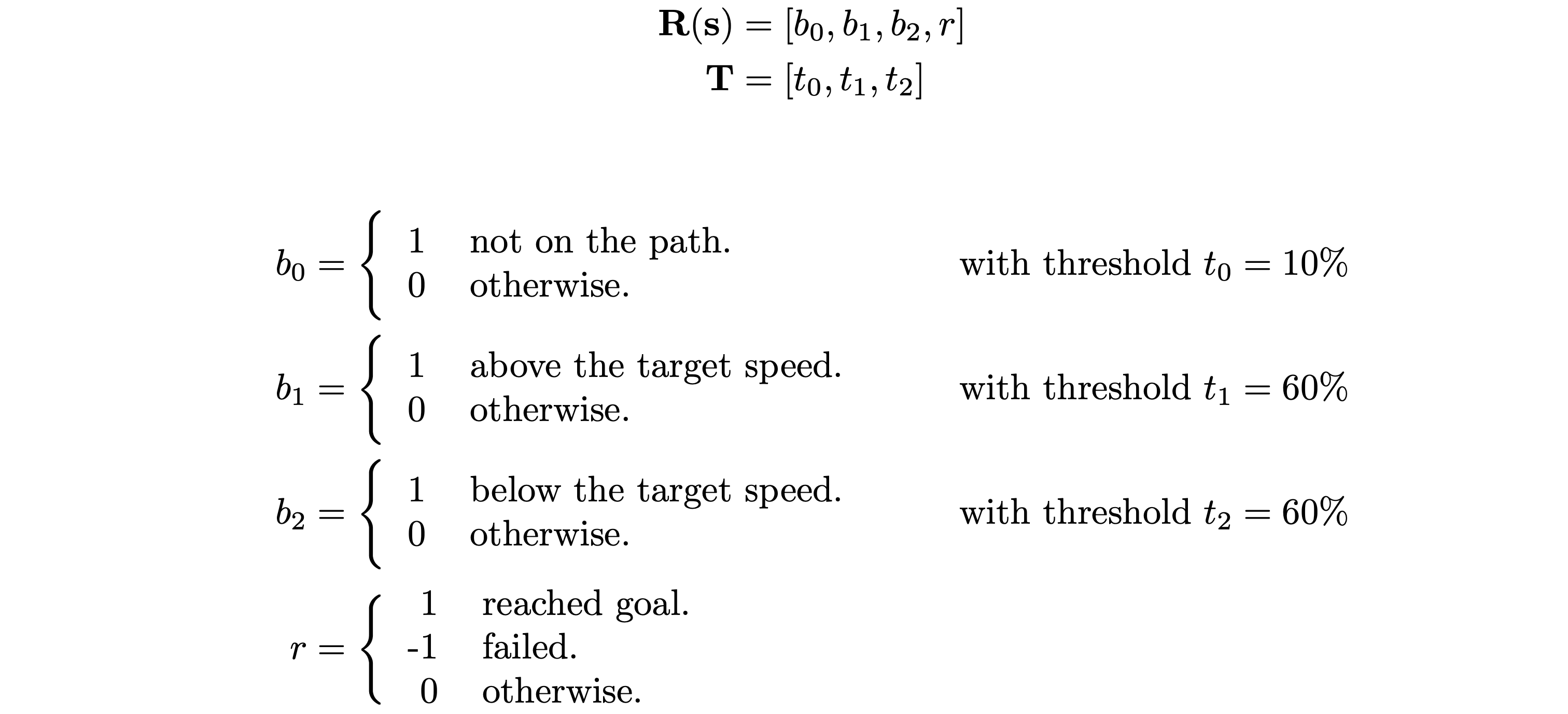We see two peculiar properties in this configuration: the two speed constraints and their high valued thresholds. Indeed, the first approach could be to set these thresholds at a lower value since we want the vehicle to be on target speed as much as possible. But the reason for both of these properties stems from the fact that it is impossible for the vehicle to be exactly on the target speed.

The speed behavior was split in two constraints because it cannot be expressed as a single constraint. For example, if the constraint were defined as:Then b1 = 1 for all time steps. The constraint’s weight would blow up and the model would get stuck trying to learn this impossible behavior.

It could be argued that the speed behavior could be defined as a single constraint like so:But defining the width of the range is equivalent to defining a threshold for the behavior. A lesser width is harder to satisfy than a larger width, as a lesser threshold is harder to satisfy than a lar ger one. However, our constraint percentages are compared to thresholds, not ranges. It is difficult to reason about a threshold value for this ranged constraint definition.

Instead, by splitting this behavior in two constraints, we’re actually splitting an equality in two inequalities. In the context of constraints and thresholds, inequalities are much easier to reason with.For the high valued thresholds t1 & t2, we start from the above identity.The first implication comes from the fact that the vehicle is 100% of the time either above or under the target speed. The second implication comes from the fact that we want our thresholds to be feasible. If their values summed to less than 100%, then the model would be trying to attain an impossible performance!

We launch the training, and now comes the time to take a deep breath, go for a nap, or get some coffee.

A few hours into training, the vehicles start driving in an unexpected way…

We realize the vehicles are in fact satisfying the constraints. The path constraint is calculated by taking the lateral distance of the vehicle to the path. The vehicle was staying on the path with respect to the path’s left-right axis, but the path constraint wasn’t giving any information to the model about the path’s front-backward axis. In fact, the model is executing an optimal behavior with this configuration: upon initialization, turn around and drive at the target speed until the episode ends.

Let’s solve this by adding a new constraint! Let’s give the model the notion of direction by simply adding a binary constraint indicating the behavior of consuming the path.Here are the results after around 20h of training with this configuration:

Pretty impressive for a simple reward function!

Lastly, the following is a great example of the effectiveness of the Reinforcement Learning paradigm. It was never explicitly specified to the model that if it spawned backwards, it should turn around. In fact, the vehicle was never prevented from driving down the path backwards. The only given metrics were the four binary constraints and the sparse reward. With this, training was able to deduce this optimal behavior.

## What next?

In this project we demonstrated that our Constrained RL framework is a promising simplified approach for training RL models for self-driving vehicles. We’ve also shown previously that it can be applied to complex NPC navigation. But these are just two of the many potential applications of this technique. Whenever specific behaviour is needed from our bots, be it for navigation, combat, or other, we believe Constrained RL can be a valuable tool!